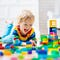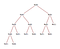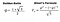# Solving the Fibonacci Sequence Using JavaScriptSunflower seeds grow in a pattern that matches the Fibonacci Sequence. image source

The Fibonacci Sequence is a something all programmers should familiarize themselves with. It is a problem that can be solved multiple ways and it can be a great intro to recursive and dynamic programming. In this blog I will solve the problem “given a number n, find the value of the nth iteration of the Fibonacci Sequence.” I will use a bottom-up solution, a recursive solution and finally a pretty neat constant time solution. All my solutions will be in JavaScript. You can click on the subject lines below to scroll to the part you’re most interested in:

History

Bottom-up Solution

Recursive Solution

Constant Time Solution

# History & Summary:

The first instances of the Fibonacci Sequence being used was in India in 200 B.C. The Fibonacci Sequence was later re-discovered by Fibonacci who wrote about it in his book Liber Abaci in 1202. He used the sequence to describe an ideal population of rabbits. A pair of rabbits are put in a field. At one month they can mate and at the end of the second month they produce another pair of rabbits. First month, one pair. Second month, still one pair. Third month, the pair produces another pair, making two pairs. Then on the fourth month, the first pair creates another, but the second pair can’t produce yet, so there were 3 pairs in total. This pattern is the Fibonacci Sequence. Written out as numbers, it goes: 1, 1, 2, 3, 5, 8, 13, 21,… etc.

There are multiple ways to solve the problem of finding the nth value of the Fibonacci Sequence. If you have not solved this problem yet, take a few minutes to try to solve it on your own.

I found one of the most confusing things about this problem was keeping track of what n represented exactly. Was I thinking of the return value as the nth iteration of the Fibonacci Sequence or as an index of an array? For a lot of problems you are fine thinking of the answers as an index to an array (first iteration is n=0 or the 0th index of an array). That is not a helpful way to think of the Fibonacci Sequence. It’s best to think of your answers as the nth iteration. The 1st iteration (n=1)will return 1. The 2nd (n=2) will also return 1. The third iteration (n=3) returns 2 and so on.

# Bottom-Up Solution:

If you tried to solve this for the first time, you may have chosen to start from the bottom and work your way to n. This is also known as a bottom-up iterative solution.

My bottom-up solution looked like this:

I start out by setting a variable sum to hold the sum and a previous sum variable prevSum. I also have a temporary storage variable, temp as I cannot just increment sum by prevSum and then set prevSum equal to sum. Since I chose to use a while loop, I also made an iteration variable i.

Then the basis case or most simple scenario is the 1st and 2nd iteration. These will both equal 1. So I set an if condition, checking if n is less than 2 and returning 1 if that’s the case. This condition is also why I can set sum and prevSum to 1. This is because I am starting from the 3rd iteration.

Then the while loop will check that I have not exceeded n, my number of Fibonacci iterations. I set temp to sum and increment sum by prevSum, then set prevSum equal to temp.

Once outside of the while loop I return sum.

This solution is fairly efficient. It has an O(n) runtime and does not use as much memory as a recursive solution.

# Recursive Solution:

Now onto recursion. Recursion can be thought of as taking a large problem and breaking it into its basic components. If these basic components are easier to solve then the larger problem, it can be a good time to use recursion.

Recursion for programmers means a function that calls itself. This method takes advantage of something called a stack. You can think about a stack as a tower of blocks.How it feels learning about stacks and recursion. image source

Each time you run a function and it returns another call of itself, a block is added to the tower. When the calls of the function returns something besides another call(the base case) and no other function calls are que’d, the function’s operation is complete. The tower of blocks is then added up by the computer and the sum of blocks returned. In the making of the iterative solution, I found the most simple cases to be any number less than 2. These cases of the 1th iteration and 2nd both return 1.

I wrote the recursive solution as follows:

As before, I checked if n is less than 2. Otherwise I returned the sum of the last 2 Fibonacci numbers. How do I know the previous Fibonacci numbers? If n is not less than 2, the previous Fibonacci numbers are a sum of its previous 2 Fibonacci numbers, or (n-1)+(n-2). Because of the stack, the computer can add all these up at the end, giving us the correct Fibonacci number.

In order to visualize a recursive function’s process, it can be helpful to make a tree. At the start of the tree, the first node is your first call of the function, for example n = 5. Each node can have 2 children nodes. This is because we call fib(n-1) and fib(n-2) each time we call the function. Each ‘leaf’ of the tree or end nodes are either fib(0) or fib(1). These are the base cases.A recursive tree plot. source

What is the runtime of this function? It can be a bit tricky to figure out. The function does little work besides calling itself. So each function call does O(1) work. That means we can calculate the runtime by counting the number of nodes on the tree we made. The top node of the tree has 2 children. Those children have 2 children, and those children have 2 children and so on. This means for any n, we should get a roughly O(2^n) runtime. It’s actually about O(1.6^n) but big o notation is only concerned with the upper limit of runtime, so this approximation of O(2^n) is adequate.

As far as space complexity or memory usage, recursive functions usually use a lot. Each function call adds another function to the stack so this implementation has an O(n) memory depth.

# Constant Time Solution:

Often problems like this will have a really cool solution. Something that you probably can’t remember for an interview, but it’s good to know that it exists. There is such a way to solve the Fibonacci Sequence in constant time or O(1). This means that no matter how large n is, we will always do the same number of operations.

I used Binet’s Formula to evaluate the Fibonacci Sequence. More info and discussion can be found here and a blog here.The Golden Ratio(left) and Binet’s Formula(right)

This is what my function looks like:

There is a downside to this method. Due to the precision of floating points, we get tiny errors. These errors get larger, the larger n is. You can read more about floating point precision here.

Thats all. I hope you enjoyed reading this. It was a lot of fun to write. Did you solve the Fibonacci Sequence slightly differently? Think you have a better solution? Feel free to leave a comment!

I write about programming and specifically CSS | HTML | JavaScript | Ruby | React.js | Redux.

## More from Jackson Beytebiere

I write about programming and specifically CSS | HTML | JavaScript | Ruby | React.js | Redux.